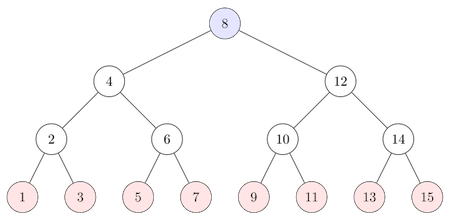Binary Search

A binary search is another way to find a desired element (again, called the "key") in a collection of elements -- which for the moment we will suppose is an array.

Unlike a linear search, to conduct a binary search the array elements must be in sorted order. (We'll assume ascending order for the purposes of our discussion.)

A binary search works by considering the middle of some range of elements in the array (initially, the entire array). One of three things could happen:

1. the middle element of the range could be the key sought, in which case one reports that position and is done;
2. the key might be less than the middle element of the range, and thus if present, must be to the left of the middle element's position; and
3. the key might be greater than the middle element of the range, and thus if present, must be to the right of the middle element's position.

In the latter two cases, we now have a smaller range to consider, so we find the new middle of this smaller range and repeat the process. If the range reduces to a single cell in the array which is not the key, then we can conclude the key sought wasn't in the array in the first place.

The following method provides an efficient implementation of a binary search.

public static int binarySearch(int[] list, int key) {
int low = 0;
int high = list.length - 1;

while (high >= low) {           //the loop only stops when
//high gets updated to something
//it shouldn't

int mid = (low + high) / 2;  //note what this does
//if (low + high) is odd

if (key < list[mid])         //update index of the
high = mid - 1;           //right-most element considered

else if (key > list[mid])    //update index of
low = mid + 1;            //left-most element considered

else
return mid;               //found it! now return the
//index and exit the method

}

//return negative value
//that doubles as an insertion
//point when turned back
//to a positive value
}

Consider the runtime analysis of such a method. As was the case with a linear search, how many comparisons we make between the key and array elements completely depends upon where the key lies in the array. If the key value is found at one of the first "middle" positions, it will be found quickly. If not, it may take a while. In the worst case, the key might be at a position that is one of the last to be used as a "middle" position -- or not be in the array at all.

To make the analysis easier, it is useful to realize that for each "middle" considered that is not the key, one's next "middle" considered will be one of two values, a value to the left and a value to the right of the current "middle".

Whatever our array looks like, we can then arrange its elements into a "tree" structure, topped by the first middle value considered, with the possible next middle values shown below it, one to the left and one to the right.

$$\begin{array}{|c|c|c|c|c|c|c|c|c|c|c|c|c|c|c|}\hline 1 & 2 & 3 & 4 & 5 & 6 & 7 & 8 & 9 & 10 & 11 & 12 & 13 & 14 & 15\\\hline \end{array}$$

As an example, consider an array filled with elements 1 to 15 in order, as shown above. Below would be the corresponding tree.The keys that represent the worst case (the ones that require the most number of "mid" values calculated) are shown in red at the bottom of the tree.

The number of comparisons needed to find keys in these worst positions corresponds to the number of levels of the tree (i.e., the depth of the tree). Here, for $n=15$ that is $4$.

Note that for an array with less than $n=2^k$ elements, the corresponding tree has at most $k$ levels. As such, searching for an element of an array using a binary search requires no more than $k = \log_2 n$ comparisons in the worst case, and is thus $O(\ln n)$ in terms of number of comparisons made.

As a binary search requires one repeatedly "jump to" the middle of a list (or sublist), this search method does not lend itself to searching for things in a linked list, where getting to the "middle node" requires visiting all of the nodes before it. However, that's not to say that we can't use a binary search when dealing with collections of "linked data" as opposed to data appearing in arrays. The trick to get the benefits of the binary search in this context is to not link the data into a list, but rather into a tree structure, not unlike the tree just drawn. We'll have more to say about this later..